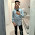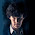## LightDo you know a longer lasting rainbow? Read below to find the answer.

### MCQs based on NCERT Chapter

Q1: Rectilinear propagation of light means,...

(a) Light can bend like curves.
(b) Light travels in straight line.
(c) Light bounces back by a medium when it falls on the medium.
(d) Light gives us sense of vision.

Q2: Shadows formation shows that light

(a) can travel in vacuum
(b) travels in straight lines
(c) exhibits wave nature.
(d) carries energy.

Q3: Images that cannot be captured on a screen are called _________.

(a) virtual images
(b) real images
(c) inverted images
(d) reflected images

Q4: Which one of the following does NOT use concave mirror?

(a) magnifier mirror by dentist
(b) reflector in flashlight
(c) solar heater
(d) rear view mirror in cars

Q5: Which of the following is NOT the other name of convex mirror?

(a) diverging mirror
(b) fish eye mirror
(c) converging mirror
(d) all of these

Q6: Which lens always gives an image which is upright, virtual and smaller than the object?

(a) concave lens
(b) convex lens
(c) both concave and convex lens
(d) none of these

Q7: When a Newton disc is rotated at good speed, the colours of the disc would blend and gives

(a) black colour
(b) white colour
(c) blue colour
(d) yellow colour

Q8: If angle of incidence of ray of light on a plane mirror is 30°, then angle of reflection is _____.

(a) 30°
(b) 45°
(c) 60°
(d) 90°

Q9: Which type of mirror is used in Periscope?

(a) convex mirror
(b) concave mirror
(c) plane mirror
(d) none of these

Q10: Primary colours (as per modern colorimetry) consists of

(a) Cyan, Magenta, Yellow
(b) Red, Green, Blue
(c) Black, Yellow, White
(d) Red, Yellow, Blue

1: (b) Light travels in straight line.
2: (b) travels in straight lines
3: (a) virtual images
4: (d) rear view mirror in cars
5: (c) converging mirror
6: (a) concave lens
7: (b) white colour
8: (a) 30°
9: (c) plane mirror
10: (b) Red, Green, Blue

Amazing Fact: A rainbow lasted for about 9 hours on 14th March, 1994, at a place called Wetherby in Yorkshire, England.1.2.3.4.This comment has been removed by the author.

5.more numericals pls

6.It is actually so use ful for grade 7 .....
Thanks for publishing these questions...
They are very useful

7.Q. 10 IS WRONG IT WILL BE RED, BLUE AND YELLOW.

1.Question is updated. As per modern mathematical colour space model it is RGB.

8.4 was also wtong

1.It is correct. Car rear mirrors are convex mirrors.

9.4 was also wtong

10.Answer 10 is wrong but question 4 is correct.
the worksheet was very helpful